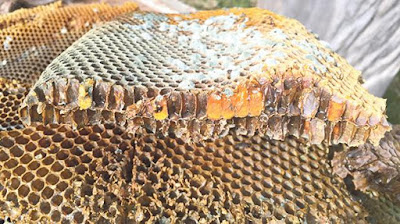## Sunday, June 03, 2018

### Can bees do maths? | Times of Malta

"The skill of tile-laying has roots dating back to at least 4000BC" according to Peter Borg and John Baptist Gauci.The two back-to-back layers of cells that compose a honeycomb. Photo: https://solarbeez.com/2016/03/12/bees-bogs-and-el-nino

Remains of Mesopotamian buildings present evidence that the Sumerians used to decorate the walls with patterns of clay tiles, generally referred to as tesserae.

The term ‘tessellation’ thus refers to the covering of a surface using one or more geometrical figures in such a way that there are no overlaps and no gaps. Restricted to two dimensions, tessellations of the plane using regular, semi-regular or irregular figures is an art that has attracted the ingenuity and creativity of many artists (see Did you know?). However, this skill is not restricted to humans. Honeybees are considered the masters of tessellations.

A polygon is regular if it has equal sides and equal angles. Only some basic mathematical knowledge is required to understand what happens if the tessellation is restricted to one where the tiles used are identical regular polygons. Combining the two well-known facts that the sum of the interior angles of a polygon with n sides is given by 2n - 4 right angles and that a whole revolution is made up of four right angles, we immediately get that the only possible values that n can take are 3, 4 and 6.

Therefore, if the plane is tessellated with identical regular polygons, then the equilateral triangle, the square and the regular hexagon are the only three possible shapes that can be availed of. But why did the honeybees decide to use regular hexagons when the other two shapes are clearly easier to construct?

Pappus, a geometer of ancient Greece who lived in the fourth century, conjectured that, apart from being aesthetically pleasing, hexagons making up the honeycomb divide a plane into shapes of equal area with the smallest total perimeter. It is thought that this conjecture actually originated in 36BC and is due to the Roman scholar Marcus Terentius Varro.

As easy as it may sound, the ‘Honeycomb Conjecture’ stood for almost two millennia before some progress was made in 1943 by László Fejes Tóth and then finally proved in 2001 by Thomas C. Hales. In other words, mathematicians proved that bees opt to build a house with no empty gaps between rooms using the tessellation requiring the least amount of wax possible among all possible tessellations of the plane (not necessarily using only one tile which is regular)!
This seems to indicate that bees solved an optimisation problem way before mathematicians did. So, can bees do maths? (See Myth Debunked.)

Did you know?
• The bottom end of each cell in a honeycomb is not flat, but has the form of a pyramid made of three rhombi. Thus, each honeycomb cell is not exactly a hexagonal prism. Honeycombs are composed of two back-to-back layers of cells, and although this configuration is more efficient than if each cell in the two layers is made up of a hexagonal prism, it is approximately two per cent less efficient than Kelvin’s structure (see Sound Bites).
• An infinite number of tiles can be devised to tessellate the plane, for instance, by starting with the square, removing a section from one side and adding it to the opposite side.